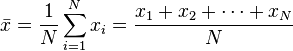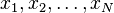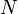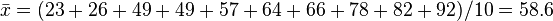# Statistics facts for kids

Kids Encyclopedia FactsScatter plots are used in descriptive statistics to show the observed relationships between different variables.

Statistics is the science of data. It enables the collection, analysis, understanding, and presentation of data. It helps in the study of many other fields, such as medicine, economics, psychology, and marketing. Someone who works in statistics is called a statistician.

Statistics provides ways to get the data needed for a study without waste, such as surveys and controlled experiments. Once the data has been collected, there are two basic ways that data can be analysed:

1. First, statistics can help describe the data. This is known as descriptive statistics. Descriptive statistics is about finding meaningful ways to summarize the data, because it is easier to use the "summary" than having to use the whole set of data all the time. Summarizing the data also allows to find common patterns. In statistics, such patterns are called probability distributions. The basic idea is to look at the results of an experiment, and look at how the results are grouped.
2. Once the results have been summarized and described they can be used for prediction. This is called Inferential Statistics. As an example, the size of an animal is dependent on many factors. Some of these factors are controlled by the environment, but others are by inheritance. A biologist might therefore make a model that says that there's a high probability, the offspring will be small in size if the parents were small in size. This model probably allows to predict the size in better ways than by just guessing at random. Testing whether a certain drug can be used to cure a certain condition or disease is usually done by comparing the results of people who are given the drug against those of people who are given a placebo.

## History

Statistics have been in use for a long time. The first known statistics are census data. The Babylonians did a census around 3500BC, the Egyptians around 2500 BC, and the Ancient Chinese around 1000 BC.

## Collecting data

Before we can describe the world with statistics, we must collect data. The data that we collect in statistics are called measurements. After we collect data, we use one or more numbers to describe each observation or measurement. For example, suppose we want to find out how popular a certain TV show is. We can pick a group of people (called a sample) out of the total population of viewers. Then we ask each one how often they watch the show, or (better) we measure it by attaching a counter to each of their television sets. For another example, if we want to know whether a certain drug can help lower blood pressure, we could give the drug to people for some time and measure their blood pressure before and after.

## Methods

Most often we collect statistical data by doing surveys or experiments. To do a survey, we pick a small number of people and ask them questions. Then, we use their answers as the data.

The choice of which individuals to take for a survey or data collection is important, as it directly influences the statistics. When the statistics are done, it can no longer be determined which individuals are taken. Suppose we want to measure the water quality of a big lake. If we take samples next to the waste drain, we will get different results than if the samples are taken in a far away, hard to reach, spot of the lake.

There are two kinds of problems which are commonly found when taking samples:

1. If there are many samples, the samples will likely be very close to what they are in the real population. If there are very few samples, however, they might be very different from what they are in the real population. This error is called a chance error (see Errors and residuals in statistics).
2. The individuals for the samples need to be chosen carefully, usually they will be chosen randomly. If this is not the case, the samples might be very different from what they really are in the total population. This is true even if a great number of samples is taken. This kind of error is called bias.

### Errors

We can reduce chance errors by taking a larger sample, and we can avoid some bias by choosing randomly. However, sometimes large random samples are hard to take. And bias can happen if different people are not asked, or refuse to answer our questions, or if they know they are getting a fake treatment. These problems can be hard to fix. See also standard error.

## Descriptive statistics

### Finding the middle of the data

The middle of the data is called an average. The average tells us about a typical individual in the population. There are three kinds of average that are often used: the mean, the median and the mode.

The examples below use this sample data:

 Name | A   B   C   D   E   F   G   H   I   J
---------------------------------------------
score| 23  26  49  49  57  64  66  78  82  92


#### Mean

The formula for the mean is$\bar x = \frac{1}{N}\sum_{i=1}^N x_i = \frac{x_1+x_2+\cdots+x_N}{N}$

Where$x_1, x_2, \ldots, x_N$ are the data and$N$ is the population size. (see Sigma Notation).

This means that you add up all the values, and then divide by the number of values.

In our example$\bar x = (23+26+49+49+57+64+66+78+82+92)/10 = 58.6$

#### Mode

The mode is the most frequent item of data. For example the most common letter in English is the letter "e". We would say that "e" is the mode of the distribution of the letters.

For example, if in a room there are 10 people with incomes of $10,$20, $20,$40, $50,$60, $90,$90, $90,$100, and $1,000,000, the mode is$90 because $90 occurs three times and all other values occur fewer than three times. There can be more than one mode. For example, if in a room there are 10 people with incomes of$10, $20,$20, $20,$50, $60,$90, $90,$90, $100, and$1,000,000, the modes are $20 and$90. This is bi-modal, or has two modes. Bi-modality is very common and often indicates that the data is the combination of two different groups. For instance, the average height of all adults in the U.S. has a bi-modal distribution. This is because males and females have separate average heights of 1.763 m (5 ft 9 + 1⁄2 in) for men and 1.622 m (5 ft 4 in) for women. These peaks are apparent when both groups are combined.

The mode is the only form of average that can be used for data that can not be put in order.

### Finding the spread of the data

Another thing we can say about a set of data is how spread out it is. A common way to describe the spread of a set of data is the standard deviation. If the standard deviation of a set of data is small, then most of the data is very close to the average. If the standard deviation is large, though, then a lot of the data is very different from the average.

If the data follows the common pattern called the normal distribution, then it is very useful to know the standard deviation. If the data follows this pattern (we would say the data is normally distributed), about 68 of every 100 pieces of data will be off the average by less than the standard deviation. Not only that, but about 95 of every 100 measurements will be off the average by less that two times the standard deviation, and about 997 in 1000 will be closer to the average than three standard deviations.

### Other descriptive statistics

We also can use statistics to find out that some percent, percentile, number, or fraction of people or things in a group do something or fit in a certain category.

For example, social scientists used statistics to find out that 49% of people in the world are males.

## Images for kidsStatistics Facts for Kids. Kiddle Encyclopedia.